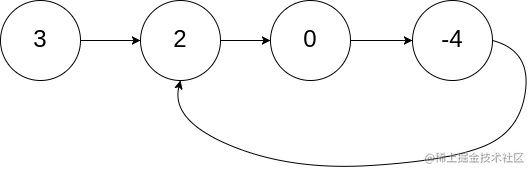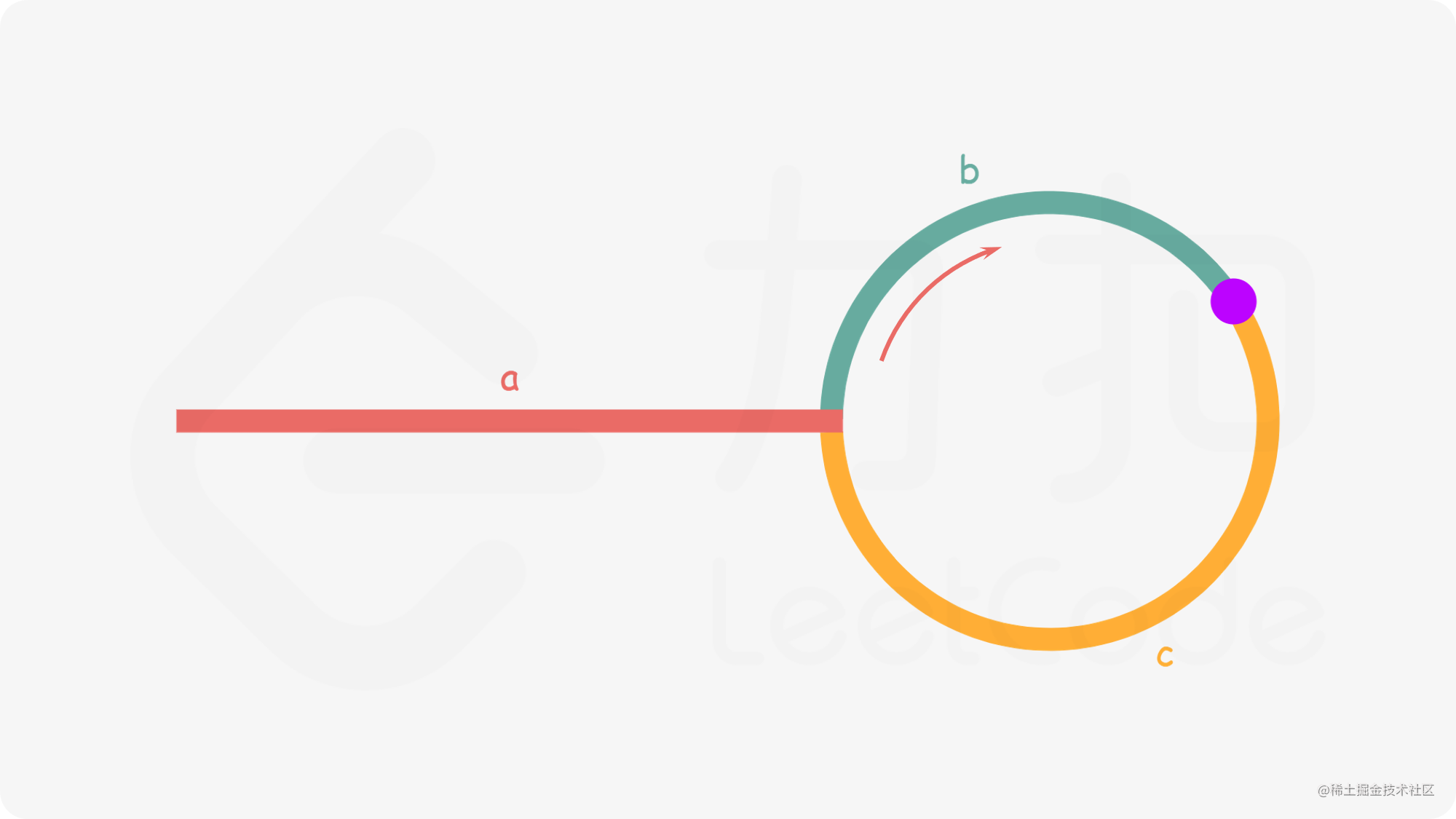# 前端算法面试必刷题系列

• 链表
• 中等

### 题目

leetcode 传送门``````输入：head = [3,2,0,-4], pos = 1

### 基本思路### 写法实现

#### 标记

``````var detectCycle = function(head) {
// 这次我们用 set
const visited = new Set()
while(cur) {
// 发现走了第二次了，说明就是入口
if (visited.has(cur)) {
return cur
}
cur = cur.next
}
return null
};

#### 快慢指针

``````var detectCycle = function(head) {
while(slow && fast && fast.next) {
slow = slow.next;
fast = fast.next.next;
if (slow === fast) {
// 新增一个节点从头开始走 当 preOther === slow 时，就是入口
while(preOther !== slow) {
slow = slow.next;
preOther = preOther.next;
}
return preOther
}
}
return null
};

## 104. K 个一组翻转链表 (reverse-nodes-in-k-group)

• 链表
• 困难

### 题目

leetcode 传送门

k 是一个正整数，它的值小于或等于链表的长度。

``````输入：head = [1,2,3,4,5], k = 2

``````输入：head = [1,2,3,4,5], k = 3

K 个一组翻转链表 拆分为

• 如何分组
• 如何翻转

### 写法实现

``````var reverseKGroup = function(head, k) {
let count = 0, cur = head;
// 获取本轮的 k 个节点(可能不够)
while(cur != null && count !== k){
cur = cur.next;
count++;
}
// 本轮满了 k 个 需要翻转
if (count === k) {
// 递归 链接后续 k个反转的链表头节点
cur = reverseKGroup(cur, k)
while(count != 0) {
// 本轮翻转
count--;
}
}
};

``````假设 k = 3
[1|]   ->   [2|]   ->   [3|]   ->   [4|] -> null

count   0            1          2           3
|                                   |

``````       [1|]   ->   [2|]   ->   [3|]   ->   [4|] -> null
|                                  ^
|__________________________________|

``````       [1|]   ->   [2|]   ->   [3|]   ->   [4|] -> null
|                                  ^
|__________________________________|
|
cur

``````       [1|]   ->   [2|]   ->   [3|]   ->   [4|] -> null
|                                  ^
|__________________________________|
|           |

``````       [1|]  <--   [2|]   ->   [3|]   ->   [4|] -> null
|          ^           ^            ^
|__________|___________|____________|
|           |

``````       [1|]  <--   [2|]   <--  [3|]   ->   [4|] -> null
|                      ^            ^
|______________________|____________|
|           |

``````
[1|]  <--   [2|]   <--  [3|]      [4|] -> null
|                      ^          ^
|______________________|__________|
|